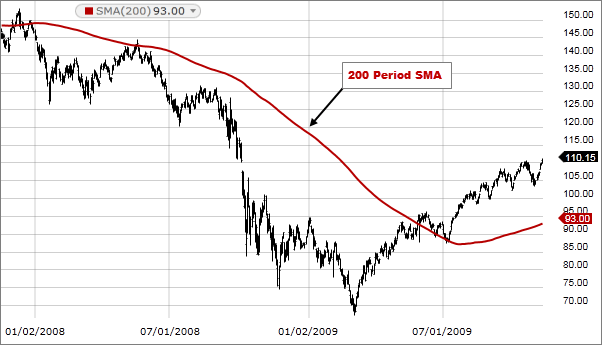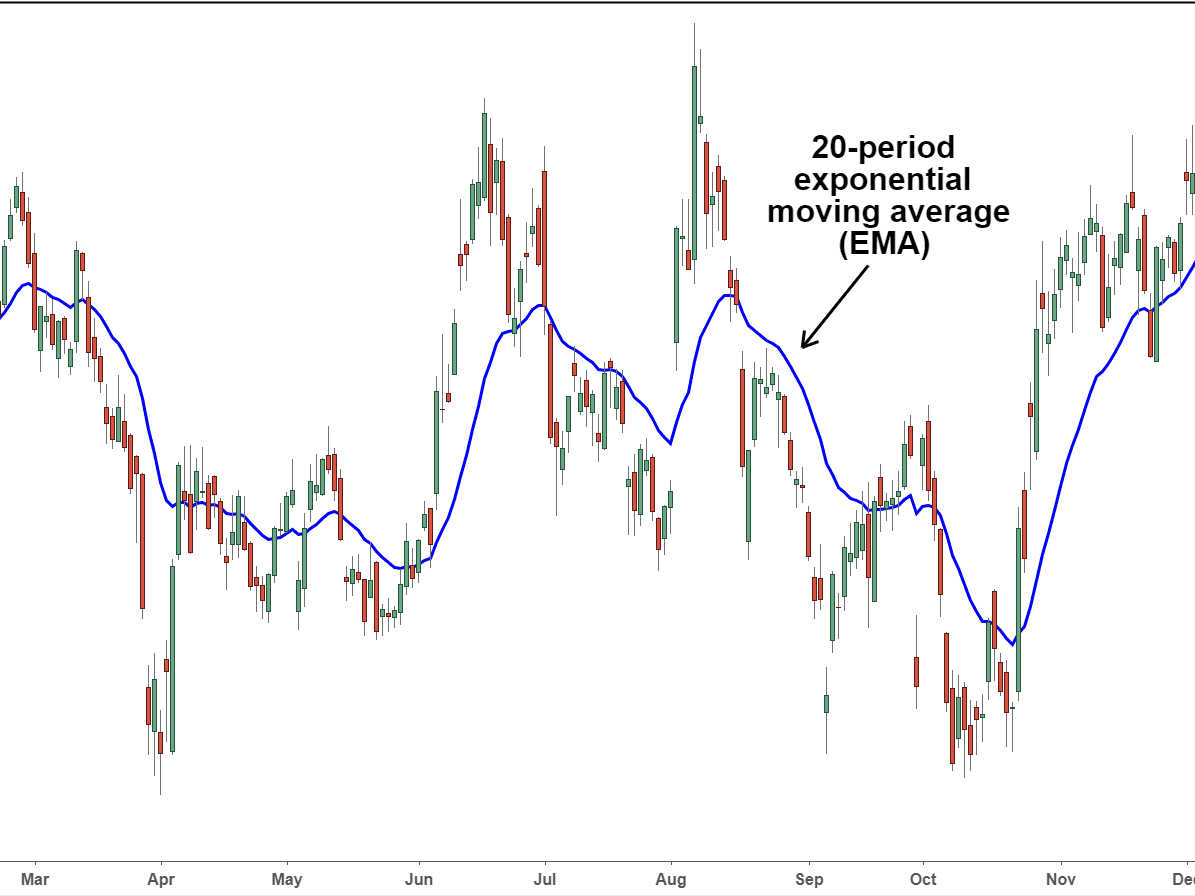# Simple Moving Average vs Exponential Moving Average

Whether you want to start trading Forex, cryptocurrencies or the stock market, one of the things that you need to know all about is the moving average. Now in case you are not sure of what moving averages are, don’t worry about it because we will explain all of this in great detail below.

With that being said, if you have no idea what moving averages are in relation to trading, then you are going to have huge problems making money. In fact, when it comes to technical analysis in trading, knowing about moving averages is one of the most important things that you need to know. Now what you also need to know is that there are different types of moving averages.

The two main types of moving averages that you need to know about are the simple moving average and the exponential moving average. Now both of these trading indicators are the same type of indicator, but they do function in slightly different ways.

Moreover, the two provide you with the same type of information, but one tends to be a little bit better in terms of recent information than the other. This is one of the main things that we will discuss today. Right now, we want to compare the simple moving average to the exponential moving average to see exactly what they are all about.

We want to figure out what they are, how they work, and what the differences between them are, so you can then figure out which of the two is best for you to use. Let’s compare the simple moving average and the exponential moving average to help make your life as a trader easier.## What are Moving Averages?

Before we can get to comparing the simple moving average to exponential moving average, what you need to know is what the moving average actually is. So in layman’s terms, this is a specific type of technical analysis indicator that is used in many different types of trading, whether it be crypto currency trading, forex trading or stock market trading.

What you also need to know is that when it comes to trading, there are four different types of indicators out there. Or in other words, indicators that are divided into four different categories and these include momentum, volatility, trend and volume indicators. What is important for you to know is that moving averages are classified as trend indicators. In other words, it helps you as a trader figure out in which direction the value of an asset or security is trending.

For instance, if there is an upward trend in a moving average, it could imply that there is a price increase on the horizon. To put it in the simplest terms, a moving average is a type of trend indicator that helps you determine in which direction of prices moving and to a certain degree will also help you determine what the momentum of that trend is.

## A Lowdown on the Simple Moving Average

Alright, so now that you know what moving averages in general are the first type that we want to take a look at is the simple moving average, and as you can probably guess by the name of it, it’s the much simpler of the two types that we are here to discuss today. The most basic definition of the SMA is that it helps to calculate the average price within a selected range or time period.

To explain this in more detail, the simple moving average takes a very specific amount of closing prices of a security or asset and then divides that number by the number of days or periods in that range. To put it in simpler terms, a simple moving average is a type of technical indicator that will help you figure out whether or not the price of an asset will continue to rise, or if it will, then reverse. To put this in other terms.

The SMA can help you determine if there is a bull or better trend on the horizon. Whether you are a stock market, trader, cryptocurrency or Forex trader, this simple moving average is a very good type of trend indicator that can inform you of the trend direction of any type of security or asset. It’s all about letting you know where and when to place those trades.First of all, let’s take a look at the various advantages that come your way. If you choose to use the SMA indicator.

• First and foremost, the simple moving average offers you a very smooth line that’s not prone to whiplashing up and down in response to slight or temporary price swings.
• What can also be said is that the SMA is best used for fairly long periods of time.

Of course, just like with any technical indicator out there, that simple moving average does also have some drawbacks that you need to be aware of.

• The number one drawback that you need to be aware of with the simple moving average is that it’s very slow to respond to recent price changes because it doesn’t put much weight on the most recent prices.
• Due to the fact that it is quite slow to respond, it doesn’t work very well for short time frames.

#### Calculating the SMA

Of course, what is important for you to know is how to actually calculate the SMA. With that being said, the simple moving average is of course the simpler of the two types that we are here to discuss today, and it’s actually quite easy to calculate. All you really need to do is to take the closing prices of a specific amount of days, say for five days, and then divide that by those five days. Take a look below for a clear example on how to do this calculation.

\$8+\$9+10+11+12 = \$50

\$50/5 = 10

## A Lowdown on the Exponential Moving Average

Now that you know what SMAs are, you need to know what exponential moving averages are. And yes, this is the more complicated of the two. The exponential moving average is another type of technical chart indicator that helps you track the price of a stock commodity, a currency pair, or anything in between.

Just like the SMA, the EMA is also a specific type of trend indicator that tells you the trend of asset or security. To a certain degree, it may also inform you of the momentum.  now with that being said there is of course a big difference between the two and the difference is that with simple moving averages the most recent prices equally weighted with all of the other prices whereas with an exponential moving average the most recent price that is used in the calculation has the most weight or importance put on it because of the fact that exponential moving averages place the most importance on the most recent price. It is therefore ideal to use for shorter time periods as it reacts very quickly to price changes. It is more accurate on short timeframes than the simple moving average.• By far the biggest advantage of the EMA is the fact that it responds very quickly to price changes, and this is useful for intraday traders.
• This type of moving average is much better at calculating short term trend reversals.
• Because the most important is given to the most recent prices, the EMA can whipsaw back and forth, or in other words, it can produce false signals due to be a little too reactionary.
• If you are engaging in long term trading then the exponential moving average indicator is not the best choice to go with.

#### Calculating the EMA

To calculate the EMA, you need to figure out the SMA for a specific period of time. For example, if you have a 20 day time period, you need to calculate the SMA for that period, and then, on the 21st day, you can use the SMA of the previous 20 days as the first EMA for yesterday.

You must then also calculate the weighting or the smoothing factor for the EMA, which is [2 / (number of observations) +1], which in this case would be [2/(20+1)] = 0.0952.

The final step of the calculation of the EMA is EMA = closing price x multiplier + EMA of previous day x (1 – multiplier). Yes, this is of course a bit complicated, but with just a little bit of practice, you should be able to master this calculation with relative ease. Just go to some price charts and give it a shot.

## The Bottom Line on the Moving Averages

Now that you know what MAs are as well as what the difference between the simple moving average and the exponential moving averages. You can start using this type of technical trend indicator to start placing profitable trades.

Remember folks, if you need help day trading, and what you need is a comprehensive education, particularly on Forex trading, then the best place to be is the Income Mentor Box Day Trading Academy. At this time, the IMB Academy is the most comprehensive, user friendly, effective, and affordable Forex trading school out there.

### CLICK BELOW TO JOIN INCOME MENTOR BOX AND START MAKING REAL PROFITS!